# Redox Reactions NEET Previous year Questions with Answer key

3. What is the change in oxidation number of carbon in the following reaction? (NEET 2020)

CH4(g) + 4Cl2(g) → CCl4(l) + 4HCl(g)

(a) + 4 to + 4                 (b) 0 to + 4

(c) – 4 to + 4                 (d) 0 to – 4

Chemistry Notes

Physics Notes

Biology Notes

4. The correct structure of tribromooctaoxide is                          (NEET 2019)

(a)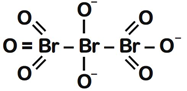(b)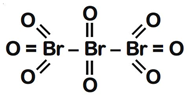(c)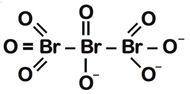(d)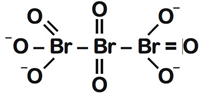### JEE-Main Practice Question paper with Solution

5.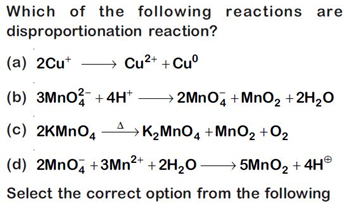(NEET 2019)

(a) (i) and (iv) only                    (b) (i) and (ii) only

(c) (i), (ii) and (iii)                     (d) (i), (iii) and (iv)

6. The oxidation state of Cr in CrO5 is                     (NEET 2019, 2014)

(a) –6                       (b) +12

(c) +6                        (d) +4

7. The correct order of N-compounds in its decreasing order of oxidation states is  (NEET 2018)

(a) HNO3, NO, N2, NH4Cl

(b) HNO3, NO, NH4Cl, N2

(c) HNO3, NH4Cl, NO, N2

(d) NH4Cl, N2, NO, HNO3

8.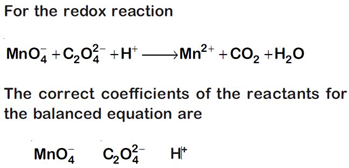(NEET 2018)

(a) 16      5             2

(b) 2        5            16

(c) 2        16           5

(d) 5       16            2

9. Hot concentrated sulphuric acid is a moderately strong oxidizing agent. Which of the following reactions does not show oxidizing behavior?  (NEET-II 2016)

(a) Cu + 2H2SO4 → CuSO4 + SO2 + 2H2O

(b) S + 2H2SO4 → 3SO2 + 2H2O

(c) C + 2H2SO4 → CO2 + 2SO2 + 2H2O

(d) CaF2 + H2SO4 → CaSO4 + 2HF

10. (I) H2O2 + O3 H2O + 2O2

(II) H2O2 + Ag2O 2Ag + H2O + O2

Role of hydrogen peroxide in the above reactions is Respectively (2014)

(a) Oxidizing in (I) and reducing in (II)

(b) Reducing in (I) and oxidizing in (II)

(c) Reducing in (I) and (II)

(d) Oxidizing in (I) and (II)

11. The pair of compounds that can exist together is                   (2014)

(a) FeCl3, SnCl2               (b) HgCl2, SnCl2

(c) FeCl2, SnCl2              (d) FeCl3, KI

### JEE-Main Practice Question paper with Solution

12. A mixture of potassium chlorate, oxalic acid and sulphuric acid is heated. During the reaction which element undergoes maximum change in the

oxidation number?                                                                       (2012)

(a) S                          (b) H

(c) Cl                         (d) C